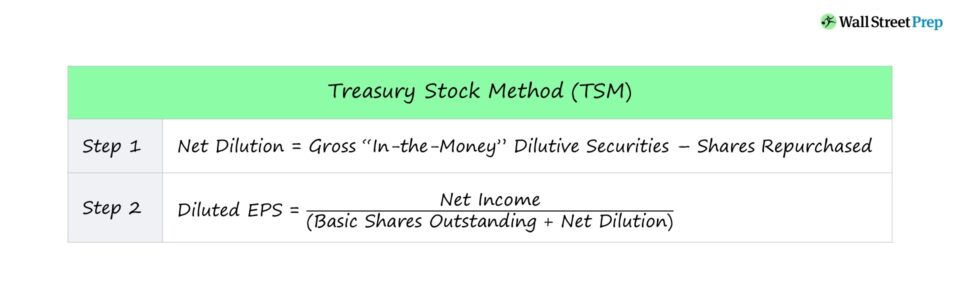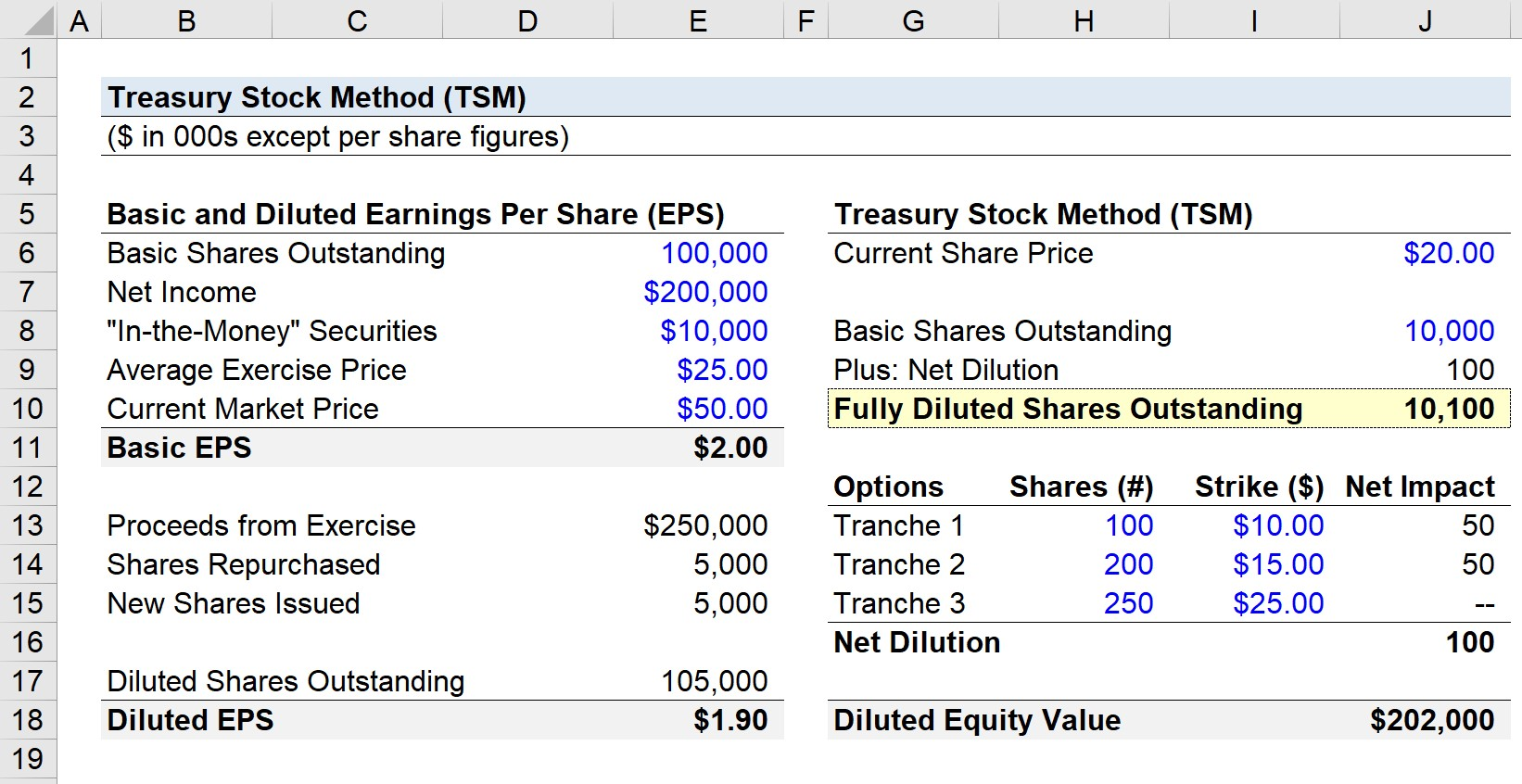Welcome to Wall Street Prep! Use code at checkout for 15% off.# Treasury Stock Method (TSM)

Guide to Understanding the Treasury Stock Method (TSM)## How to Calculate Treasury Stock Method (TSM)?

Under the treasury stock method (TSM) approach, the total diluted share count takes into account the new shares issued by the exercising of options and other dilutive securities that are “in-the-money” (i.e., the current share price is greater than the exercise price of the option/warrant/grant/etc.).

Conceptually, the treasury stock method (TSM) approximates what a company’s earnings per share (EPS) would be under the assumption that its dilutive securities, such as options, are exercised.

Another key assumption inherent to the TSM is that the (typically cash) proceeds from the exercising of these dilutive securities (i.e., option proceeds) are then used to repurchase shares under the belief that a rational company would attempt to mitigate the dilutive impact of the options by doing so.

Unlike the calculation of the basic share count and coinciding basic earnings per share (EPS), metrics based on diluted shares outstanding also consider a company’s dilutive securities such as options, rather than just the basic shares.

Hence, the fully diluted shares outstanding count is a relatively more accurate representation of the actual equity ownership and equity value per share of a company.

The exclusion of these types of securities into common equity would mistakenly inflate the earnings per share (EPS) figure.

## Treasury Stock Method Formula (“If Converted”)

The formula for the total diluted share count consists of all basic shares, as well as the new shares from the hypothetical exercise of all in-the-money options and conversion of convertible securities.

Net Dilution = Gross “In-the-Money” Dilutive SecuritiesShares Repurchased

Here, the number of shares repurchased is equal to the option proceeds (the number of gross “in-the-money” dilutive securities multiplied by the strike price) divided by the current share price.

Besides options, other examples of dilutive securities include warrants and restricted stock units (RSUs).

• Warrants: Similar financial instruments to options but result in the issuance of new shares if exercised
• Restricted Stock Units (RSUs): Issued to the company’s management team with a convertible feature attached.

If disclosed, options are evaluated on a tranche-by-tranche basis to determine if they are “in the money.”

Each tranche has a strike price, which the option holder must pay in order to exercise the option as part of the contractual agreement.

• “In-the-Money” Options ➝ Strike Price < Current Share Price
• “At-the-Money” Options ➝ Strike Price = Current Share Price
• “Out-of-the-Money” Options ➝ Strike Price > Current Share Price

Furthermore, the EPS formula divides the net income of a company by its share count, which can be either on a basic or diluted basis.

That said, if a company has issued such securities in the past (i.e., the potential for conversion), its diluted EPS in all likelihood is going to be lower than its basic EPS.

The reason is that the denominator (the share count) has increased, whereas its numerator (net income) remains constant.

Diluted EPS = Net Income ÷ (Basic Shares Outstanding + Net Dilution)

In terms of the steps involved in the TSM, first, the number of in-the-money options and other dilutive securities are summed up, and that figure is then added to the number of basic shares outstanding.

Note that only the securities deemed “in-the-money” are assumed to have been exercised, therefore those “out-of-the-money” are not included in the new share count.

In the subsequent step, the TSM assumes the entirety of the proceeds from the exercising of those dilutive options goes towards repurchasing stock at the current market share price. The assumption here is that the company would repurchase its shares in the open market in an effort to reduce the net dilutive impact.

## Insider’s Tip: Exercisable vs Outstanding Options

Companies oftentimes disclose both their “outstanding” and “exercisable” options, since certain outstanding options will have yet to vest.

For a long time, it was considered standard to include only the number of options and dilutive securities that are exercisable in the calculation of diluted shares, as opposed to outstanding.

However, the case could be made that in order to be more conservative in the diluted share count calculation, the number of outstanding options should actually be used despite not all being exercisable on the date of the valuation. This is done to reflect the reality that the majority of unvested options have a high probability of vesting someday, which is a practice that investors and firms have increasingly adopted in recent years.

Another minor complexity to be aware of is the tax benefit stemming from the elimination of interest expense or dividends associated with convertible securities (e.g., convertible debt) and preferred equity.

## Treasury Stock Method Calculator (TSM)

We’ll now move to a modeling exercise, which you can access by filling out the form below.Submitting...

## 1. TSM Operating Assumptions

Suppose a company has 100,000 common shares outstanding and \$200,000 in net income in the last twelve months (LTM).

• Common Shares Outstanding = 100,000
• LTM Net Income = \$200,000

If we were calculating the basic EPS, which excludes the impact of dilutive securities, the EPS would be \$2.00.

• Basic EPS = 200,000 ÷ 100,000 = \$2.00

But since we must account for the ITM securities not yet exercised, we multiply the potential shares issued by the average exercise price to get the total proceeds assuming they were exercised by the holder, which we calculate as \$250,000 (10,000 multiplied by the exercise price of \$25.00).

Upon dividing the exercise proceeds of \$250,000 by the current market share price of \$50.00, we get 5,000 as the number of shares repurchased.

We can then subtract the 5,000 shares repurchased from the 10,000 new securities created to arrive at 5,000 shares as the net dilution (i.e., the number of new shares post-repurchase).

• Net Dilution = 5,000
• Diluted Shares Outstanding = 105,000

## 2: Diluted Earnings per Share Calculation (EPS)

After dividing the net income of \$200,000 by the diluted share count of 105,000, we arrive at a diluted earnings per share (EPS) of \$1.90.

• Diluted Earnings per Share (EPS) = \$200,000 ÷ 105,000 = \$1.90

In comparison to our starting point, the basic EPS of \$2.00, the diluted EPS is \$0.10 less.

## 3. Treasury Stock Method Calculation Example (TSM)

Suppose we were just given two assumptions for our illustrative exercise:

• Current Share Price = \$20.00
• Basic Shares Outstanding = 10mm

If we were to ignore the dilutive impact of non-basic shares in the calculation of equity value, we would arrive at \$200mm.

But since we are accounting for the impact of potentially dilutive securities, we must calculate the net impact from in-the-money options. Here, we have three different tranches of options.

• Tranche 1: 100mm Potential Shares; \$10.00 Strike Price
• Tranche 2: 200mm Potential Shares; \$15.00 Strike Price
• Tranche 3: 250mm Potential Shares; \$25.00 Strike Price

The formula for calculating the net dilution from each tranche of options contains an “IF” function that first confirms that the strike price is less than the current share price.

If the statement is true (i.e., the options are exercisable), then the associated number of potential new shares issued is output.

Here, the current share price exceeds the strike price for the first two tranches (\$10 and \$15) but not the third tranche (\$25).

Considering how TSM assumes the company repurchases shares at the current share price, thus the strike price is multiplied by the number of potential new shares, before being divided by the current share price.

## 4. Treasury Stock Method Analysis (TSM)

The difference between the number of shares assumed to have been issued related to the dilutive securities and the number of shares repurchased as part of TSM is the net dilutive impact.

In the last part of the formula, the number of shares repurchased is deducted from the total potential shares issued in order to calculate the net dilution, which is completed for each of the three option tranches.

By multiplying the fully diluted shares outstanding by the current share price, we calculate that the net impact of dilutive securities is \$2mm and the diluted equity value is \$202mm.Step-by-Step Online Course

### Everything You Need To Master Financial Modeling

Enroll in The Premium Package: Learn Financial Statement Modeling, DCF, M&A, LBO and Comps. The same training program used at top investment banks.

Inline Feedbacks